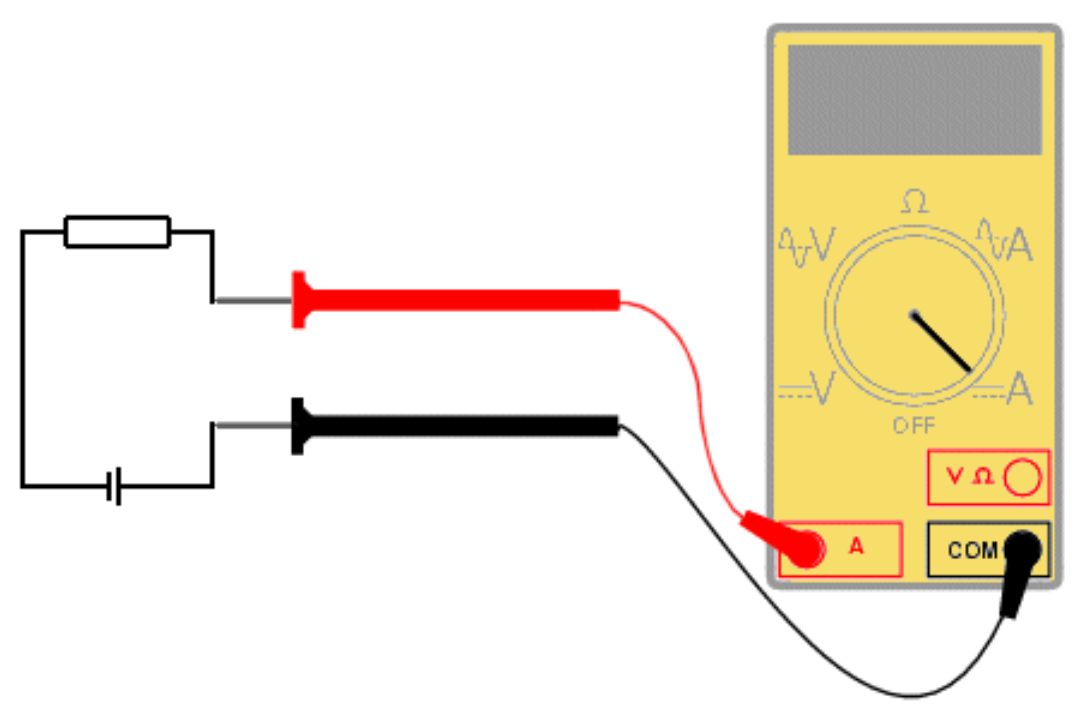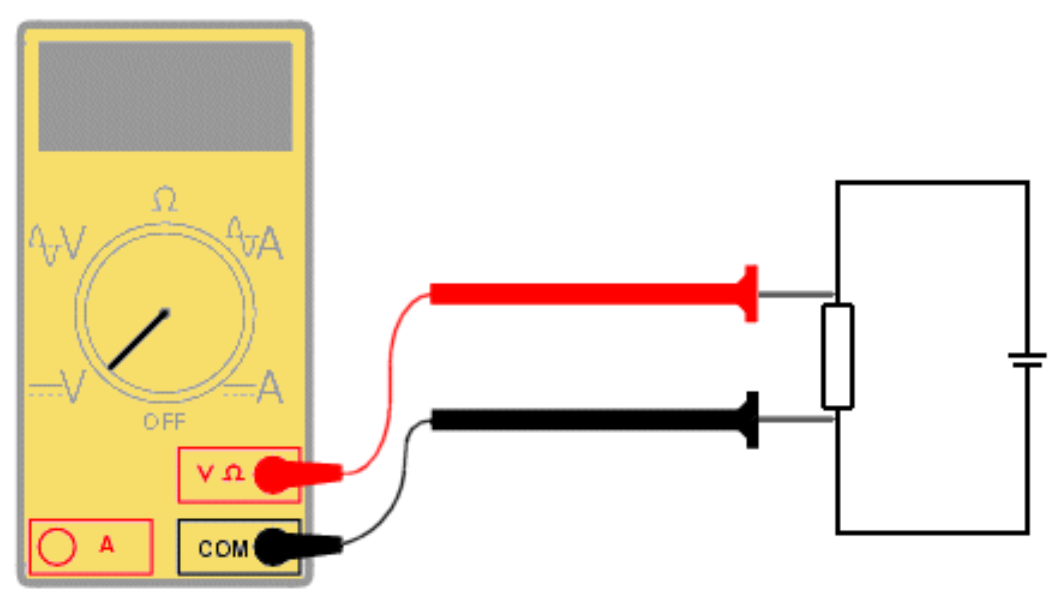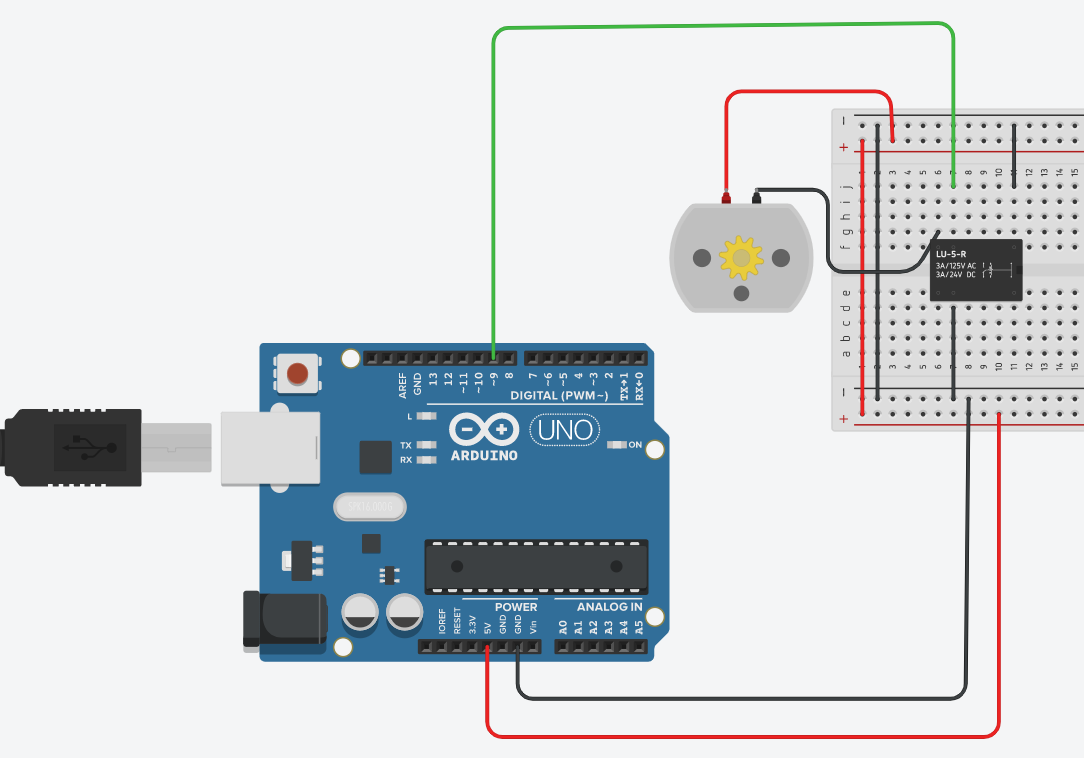Group  Assignment

Measure the power consumption of an output device.

CurrentSERVO MOTOR POWER MEASURE

• now, lets test the values from operating the servo with rotating 13- degree in both direction:

• reading are still funny, and noisy, let's try to lower the moving speed and the load on the servo:

• ok, more stable, so the servo is using 4.4 to 4.8 volt to rotate , let's take an average of 4.6 volt. What about current, when measuring current make sure that you are connecting the multimeter in the right way.. Series

• From the start, with low load, the current is 0.08 Amp and then the servo load increases, the current reaches up to 0.30 Amp. With that, we can measure the power the servo use:low load: avg V = 4.6 and min amp = 0.08 A >>>>> so the power = 4.6 X 0.08 =0.368 W

• high load : avg V X max amp =0.3A >>>>> then power = 4.6 X 0.3 = 1.38 W

RELAY POWER CONTROL MEASURE to run DC MOTOR ON OR OFF

here is the circuit connection:Keep in touch

TalentS Lab is owned by National Talents Company